Next: Exercises Up: MarkovChain_9_18 Previous: Markov Chains

Regular Markov Chain

An square matrixis called regular if for some integerall entries ofare positive.

Example

The matrix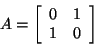is not a regular matrix, because for all positive integer,The matrix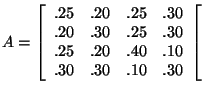is a regular matrix, becausehas all positive entries.

It can also be shown that all other eigenvalues of A are less than 1, and algebraic multiplicity of 1 is one.

It can be shown that ifis a regular matrix thenapproaches to a matrix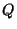whose columns are all equal to a probability vectorwhich is called the steady-state vector of the regular Markov chain.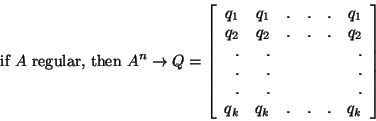where.

It can be shown that for any probability vector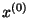whengets large,approaches to the steady-state vector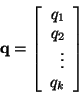.

That is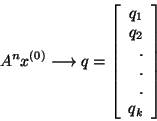where.

It can also be shown that the steady-state vector q is the only vector such thatNote that this shows q is an eigenvector of A andis eigenvalue of A.

SubsectionsNext: Exercises Up: MarkovChain_9_18 Previous: Markov Chains
Ali A. Daddel 2000-09-18